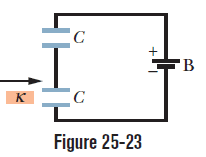# Capacitors in a series circuit W/ one dielectric

• Nemo_Sol

#### Nemo_Sol

Problem: When a dielectric slab is inserted between the plates of one of the two identical capacitors in Fig. 25-23, do the following properties of that capacitor increase, decrease, or remain the same: (a) capacitance, (b) charge, (c) potential difference (d) How about the same properties of the other capacitor?

CAPACITOR 1 = CAPACITOR WITH DIELECTRIC |CAPACITOR 2 = CAPACITOR WITHOUT DIELECTRIC(ABOVE CAPACITOR 1 IN THE DIAGRAM)

I said that the potential of the first capacitor decreases and that the charge it stores also increases. For the 2nd capacitor, I said it's capacitance would decrease. I'm not so sure though, I think it may stay the same as well?
Potential would increase and charge would increase for the 2nd capacitor as well
.Attempt at Solution: The main problem I'm having at solving this is the fact that both charge and voltage for the individual capacitors are variable. Please explain the situation and why the values for potential, capacitance and charge either decrease, increase or stay the same.

What I think: When a dielectric is added, E between the capacitor decreases by a factor of k so voltage must decrease for the first capacitor and thus the voltage for the 2nd capacitor must increase by the same amount to fulfill Kirchhoff's laws.

Adding a dielectric also allows for a capacitor to store more charge at the same potential so the first capacitor must store more charge since c = q/v <- direct relationship.

I'm confused on what happens to the 2nd capacitor. They're in series so there's that inverse relationship and total capacitance decreases.